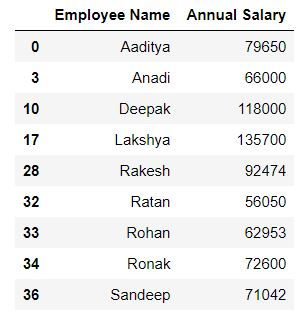top of page
Search
• Ekta Aggarwal

# Filtering rows and columns in a dataframe

In this data we will learn how to filter rows and columns in a dataframe using loc, iloc, np.where and pd.query function!

### Difference between loc and iloc

When you filter the data using loc: You need to provide either column or index names or a boolean vector.

While on filtering the data using iloc you need to provide positions (indices) of the columns or index to be fetched.

### Dataset:

In this tutorial we will make use of following CSV file:

Employee_info
.csv

Let us read our file using pandas' read_csv function. Do specify the file path where your file is located:

```import pandas as pd

### Filtering with loc

To filter the data with loc we need to provide the column or index names!!!!!

Basic sytax for filtering:

For filtering the data we need to provide 2 positions separated by a comma inside a comma i.e .[ a,b] where a denotes the row names or Boolean vector for rows, while b is the column names or Boolean vector for columns. To select all the rows or all the columns we denote it by a colon ( : )

Task: Filter the data for all the rows and 2 columns: Employee name and gender.

Since we are selecting all the rows thus we have written a colon ( :) instead of 'a'.

To select multiple columns we have providing our list ['Employee Name','Gender'] in place of 'b'

`mydata.loc[:,['Employee Name','Gender']]`

Task: Filter the data for first 5 rows and 2 columns: Employee name and gender.

Since we are selecting first 5 rows only thus we have defined our range 0:5 in place of 'a' which means start from row 0 till row 4 (5 is excluded)

`mydata.loc[0:5,['Employee Name','Gender']]`

Alternatively

range(5) means 0:5 (i.e. 5 is excluded)

`mydata.loc[range(5),['Employee Name','Gender']]`Task: Filter the data for first 5 rows and all the columns

`mydata.loc[range(5),:]`

### Filtering for consecutive columns:

To select consecutive columns we can write columnName1 : columnNameN by which all columns starting from columnName1 till columnNameN will be selected.

Task: Filter first 5 rows and columns from employee name till annual salary.

Here we have written "Employee Name" : "Annual Salary " to retrieve all the column between them (including both of them)

`mydata.loc[range(5),"Employee Name" : "Annual Salary "]`

### Filtering using Boolean vectors.

Task: Filter the data for the rows where gender is 1.

In the below code mydata.Gender == 1 returns a Boolean vector which takes the value True when gender is 1, otherwise False.

Wherever our Boolean vector has True, only those rows will be selected.

`mydata.loc[mydata.Gender == 1,:]`

### Filtering for multiple conditions

AND condition - when multiple condition need to be true

Task: Filter the data for the rows where gender is 1 and department is consulting.

In the below code (mydata.Gender == 1) & (mydata.Department == 'Consulting') returns a Boolean vector which takes the value True when both gender is 1 and Department is consulting, otherwise False.

`mydata.loc[(mydata.Gender == 1) & (mydata.Department == 'Consulting'),:]`Task: In the above query select only employee name and salary

`mydata.loc[(mydata.Gender == 1) & (mydata.Department == 'Consulting'),['Employee Name','Annual Salary ']]`OR condition - when at least one condition needs to be true

Task: Filter the data for the rows where either gender is 1 or Salary is more than 110000.

Since we need to apply an 'or' condition i.e. at least one of the conditions should be true thus in Python we use '|' to denote 'or'

`mydata.loc[(mydata.Gender == 1) | (mydata['Annual Salary '] > 110000),['Employee Name','Annual Salary ']]`

IN CONDITION

Task: Filter for rows where department is either consulting or outsourcing.

We can achieve this using or '|' condition as follows:

`mydata.loc[(mydata.Department == 'Outsourcing') | (mydata.Department == 'Consulting'),:]`

But suppose we have to choose for multiple values (say 10 departments) then writing OR condition would be too tedious.

To simplify it we have .isin( ) function in Python. Inside isin( ) we specify the list of values which need to be searched.

In the following code we have written mydata.Department.isin(['Outsourcing','Consulting']) i.e. this will return a Boolean vector where department name is either outsourcing or consulting.

`mydata.loc[mydata.Department.isin(['Outsourcing','Consulting']),:]`

### Excluding the rows satisfying a particular condition

Task: Filter for rows where department is neither consulting nor outsourcing.

It means we do not want the department names to be consulting and outsourcing. To do this, we can firstly create a vector using .isin( ) which will return True where department is consulting or outsourcing, and then specify to exclude such rows i.e. provide negation.

To provide a negation we write a (~) symbol before the Boolean vector.

`mydata.loc[~mydata.Department.isin(['Outsourcing','Consulting']),:]`### pd.query

We can filter our rows using pd.query( ) function

Task: Filter for rows where Gender is 1.

`mydata.query('Gender ==1')`

### Filtering with iloc

To filter the data with iloc we need to provide the column or index names!!!!!

Task: Filter for first 5 rows. (Since our index names are 0:44, thus iloc and loc lead to same output)

`mydata.iloc[range(5)]`Task: Filter for first 5 rows and first 3 columns.

To filter for first 3 columns we have defined 0:3 after comma.

`mydata.iloc[0:5,0:3]`### Obtaining indices using np.where

Task: Filter for rows where department is consulting.

Numpy's where function returns the indices wherever a particular condition is found True. We can then use those indices in iloc to filter the rows.

`import numpy as np`
`np.where(mydata['Department'] == 'Consulting')`

Output:

(array([ 0, 2, 3, 7, 8, 10, 17, 19, 21, 25, 28, 31, 32, 33, 34, 36, 41], dtype=int64),)

`mydata.iloc[np.where(mydata['Department'] == 'Consulting')]`bottom of page